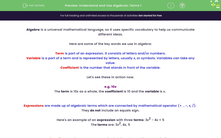# Understand and Use Algebraic Terms 1

In this worksheet, students will learn the difference between key algebraic concepts: term, expression, equation and formula, plus touch on the concept of identity.Key stage:  KS 4

Year:  GCSE

GCSE Subjects:   Maths

GCSE Boards:   Pearson Edexcel, OCR, Eduqas, AQA,

Curriculum topic:   Algebra

Curriculum subtopic:   Notation, Vocabulary and Manipulation Algebraic Formulae

Popular topics:   Algebra worksheets

Difficulty level:#### Worksheet Overview

Algebra is a universal mathematical language, so it uses specific vocabulary to help us communicate different ideas.

Here are some of the key words we use in algebra:

Term is part of an expression. It consists of letters and/or numbers.

Variable is a part of a term and is represented by letters, usually x, or symbols. Variables can take any value.

Coefficient is the number that stands in front of the variable.

Let's see these in action now.

e.g. 10x

The term is 10x as a whole, the coefficient is 10 and the variable is x

Expressions are made up of algebraic terms which are connected by mathematical operator (+  , -, x, /).

They do not include an equals sign.

Here's an example of an expression with three terms: 3x2 - 4x + 5

The terms are: 3x2, 4x, 5

3x24y+2

Equations are the same as expression but they do contain an equals sign.

As a result of this, we can solve them for some, but not all, values of x (or another variable).

This is an equation: 5x + 9 = 24

And so is this: x2 + 3x - 12 = 0

Formula is related to an equation.

Formulae will also have an equals sign, but they will be true for many values of the variable.

Therefore, we cannot solve a formula, unless we know the values of some of the variables

You might have seen some formulae in maths or science already.

Some well-known examples include:

Formula for speed: s = d ÷ t

Formula for area of a circle: A = πr2

To use these formulae, you need to know the value of one (or more of the variables).

In this activity, we will identify where these definitions apply and, therefore, which calculations we can and cannot perform in each situation.

### What is EdPlace?

We're your National Curriculum aligned online education content provider helping each child succeed in English, maths and science from year 1 to GCSE. With an EdPlace account you’ll be able to track and measure progress, helping each child achieve their best. We build confidence and attainment by personalising each child’s learning at a level that suits them.

Get started#### Popular Maths topics

••••